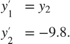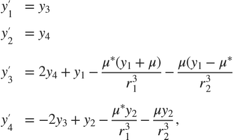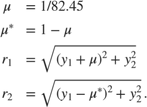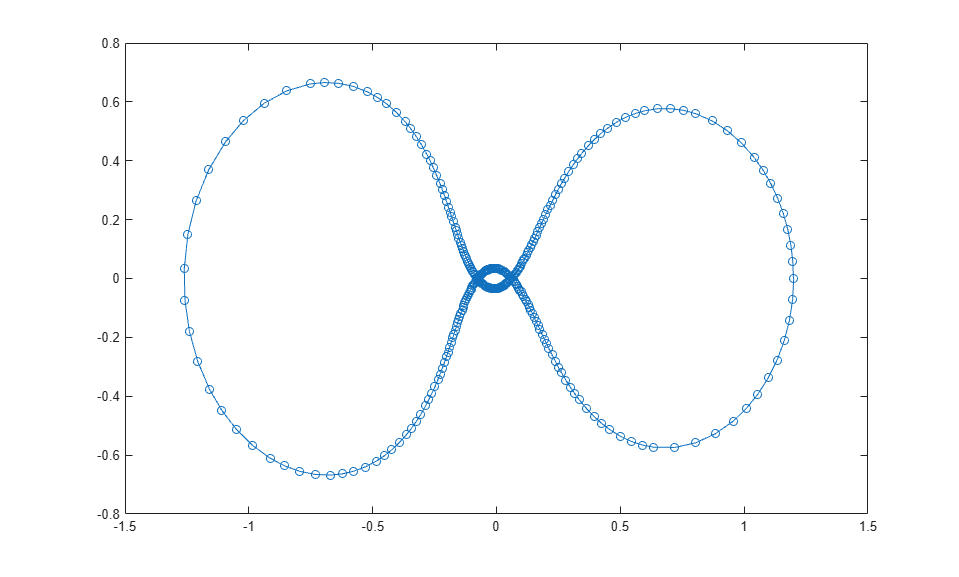## ODE 事件位置

### 编写事件函数

[value,isterminal,direction] = myEventsFcn(t,y)

ode15i 的情况下，事件函数还必须接受 yp 的第三个输入参数。

• value(i) 是描述第 i 个事件的数学表达式。当 value(i) 等于零时发生事件。

• 如果当第 i 个事件发生时停止积分，则 isterminal(i) = 1。否则为 0

• 如果需要查找全零值（默认值），则 direction(i) = 0。值为 +1 时仅在事件函数递增的位置查找零，值为 -1 时仅在事件函数递减的位置查找零。指定 direction = [] 将对所有事件使用默认值 0

$y\text{'}\text{'}=-1+y{\text{'}}^{\text{\hspace{0.17em}}2}\text{\hspace{0.17em}},$

function [position,isterminal,direction] = appleEventsFcn(t,y) position = y(1); % The value that we want to be zero isterminal = 1; % Halt integration direction = 0; % The zero can be approached from either direction end

### 事件信息

[t,y,te,ye,ie] = odeXY(odefun,tspan,y0,options)

• te 是发生事件的时刻的列向量。

• ye 包含 te 中的每个事件时刻对应的解值。

• ie 包含事件函数返回的向量的索引。这些值指示求解器检测到的事件。

sol = odeXY(odefun,tspan,y0,options)

### 局限性

ODE 求解器采用的与事件函数配合使用的求根机制存在下列局限性：

• 如果在积分的第一步即发生终止事件，则求解器会将该事件记录为非终止事件并继续积分。

• 如果在第一步发生多个终止事件，则仅记录第一个事件，并且求解器会继续积分。

• 零值由每步之间的符号交叉确定。因此，对于两步间有偶数个交叉的函数而言，可能会错失其零值。

### 简单事件位置：弹球function [value,isterminal,direction] = bounceEvents(t,y) value = y(1); % Detect height = 0 isterminal = 1; % Stop the integration direction = -1; % Negative direction only 

ballode### 高级事件位置：限制性三体问题orbitode.m 中嵌套的事件函数将搜索两个事件。一个事件查找距离起点最远的点，另一个事件查找宇宙飞船返回到起点的点。即使积分器使用的步长并非通过事件位置确定，也会准确定位事件。在此示例中，指定过零方向的功能非常重要。返回到起点的点和距离起点最远的点具有相同的事件值，并由交叉方向来区分这两个点。为此行为编码的事件函数为

function [value,isterminal,direction] = orbitEvents(t,y) % dDSQdt is the derivative of the equation for current distance. Local % minimum/maximum occurs when this value is zero. dDSQdt = 2 * ((y(1:2)-y0(1:2))' * y(3:4)); value = [dDSQdt; dDSQdt]; isterminal = [1; 0]; % stop at local minimum direction = [1; -1]; % [local minimum, local maximum] end 

orbitode 
This is an example of event location where the ability to specify the direction of the zero crossing is critical. Both the point of return to the initial point and the point of maximum distance have the same event function value, and the direction of the crossing is used to distinguish them. Calling ODE45 with event functions active... Note that the step sizes used by the integrator are NOT determined by the location of the events, and the events are still located accurately.